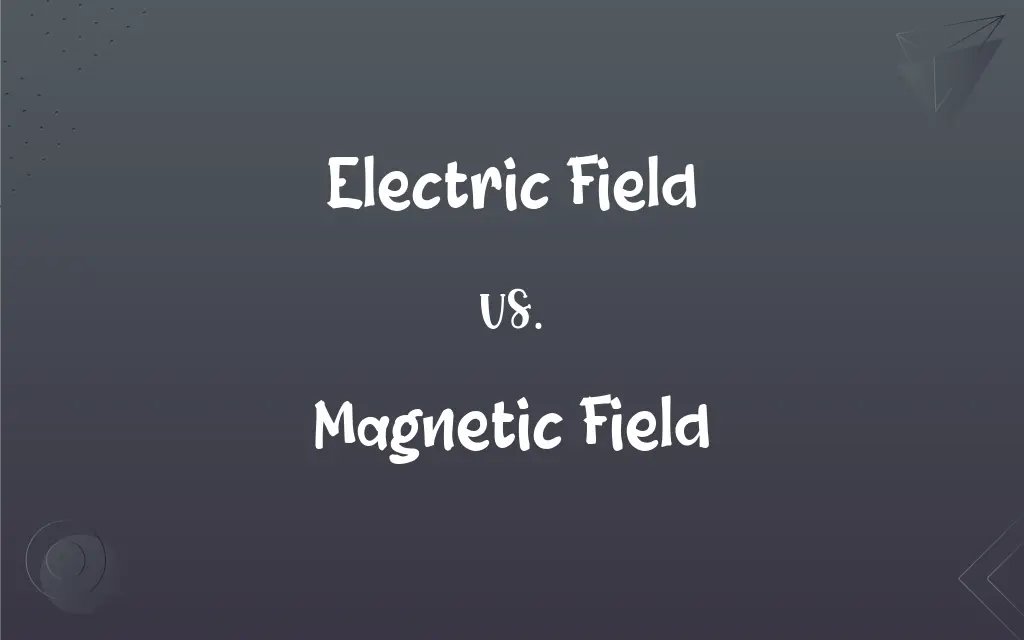# Electric Field vs. Magnetic Field

The main difference between the electric field and the magnetic field is that electric field produced around the static charge particles which are either negative or positive, whereas the magnetic field is an area exerted around the magnetic force obtained by moving electric charges.## Key Differences

Both electric and magnetic fields are vector quantities as they have magnitudes and directions.
The electric field’s SI unit is Newton/Coulomb, whereas the SI unit of the magnetic field is Tesla.
The electric field may be monopole or dipole while the magnetic field is the only dipole.
In the electric field, electromagnetic field generates VARS(Capacitive), on the contrary, in the magnetic field, electromagnetic field absorbs VARS(Inductive).
The electric field does not form a closed loop while the magnetic field forms a closed loop.
Electric field produced around the static charge particles which is either negative or positive, whereas the magnetic field is an area exerted around the magnetic force obtained by moving electric charges.
An electrometer measures the electric field; in contrast, the magnetometer measures the magnetic field.
Both electric and magnetic field creates an “electromagnetic field,” and the movement of the electric field in an electromagnetic field is perpendicular to the magnetic field whereas the movement of the magnetic field in an electromagnetic field is perpendicular to the electric field.
The force that the electric field produces is proportional to the electric charge whereas force produced by the magnetic field is proportional to charge and speed of electric charge.

## Comparison Chart

### .

It is the force around the static electrical charge particles.
The region around the magnetic where poles exhibit a force of attraction or repulsion by moving of electric charges is called a magnetic field.

### Unit

Volt/meter or Newton/coulomb
Tesla (Newton*Second)/(Coulomb*Meter)

E
B

### Formula

E=q/F​=1/4πϵ0.​​r2/qi​​r^
B= 2πr/μ0​I​

### Pole

Monopole or dipole.
Dipole.

### Movement in the Electromagnetic Field

Perpendicular to the magnetic field.
Perpendicular to the electric field.

### Electromagnetic Field

Generates VARS(Capacitive)
Absorbs VARS(Inductive)

### Forces

Proportional to the electric charge.
Proportional to charge and speed of electric charge

Electrometer
Magnetometer

Vector
Vector

### Type of Charge

Negative or positive charge.
North or South pole.

### Dimension

Exist in two dimensions.
Remain in three dimensions.

### Loop

Do not form a closed loop.
It forms a closed loop.

### Work

It can do work(speed and direction of particle charges).
It cannot do work(speed of particles remain constant).

### Electric Field vs. Magnetic Field

The electric field produced around the static charge particles which are either negative or positive, whereas the magnetic field is an area exerted around the field having a magnetic force by the moving of electric charges. The electric field does not rest on the magnetic field, and the same as the magnetic field does not depend on the electric field. In the electric field, electromagnetic field generates VARS(Capacitive), on the contrary, in the magnetic field, electromagnetic field absorbs VARS(Inductive). The electric field may be monopole or dipole while the magnetic field is the only dipole. The force that the electric field produces is proportional to the electric charge whereas force produced by the magnetic field is proportional to charge and speed of electric charge. The electric field does not form a closed loop while the magnetic field forms a closed loop. The unit of the electric field is volt/meter or newton/coulomb, whereas the unit of the magnetic field is Tesla. The electric field is denoted by E, whereas B denotes the magnetic field.

### What is Electric Field?

The force around the static electrical charge particles, either positive or negative, is called the electric field. An electric field happens wherever a voltage is existing. The electric field generates around appliances and wires where a voltage exists. The electric field has magnitude and direction. So that’s why it is a vector quantity. E symbolizes the electric field. The unit of the electric field is Volt/meter or Newton/coulomb. The strength of an electric field decreases as we move away from the source. It may exist independently like in the absence of a magnetic field; an electric field exists in the form of static electricity. Both electric and magnetic field creates an “electromagnetic field,” and the movement of the electric field in the electromagnetic field is perpendicular to the magnetic field. In the electric field, the electromagnetic field generates VARS(capacitive). The electric field may be monopole or dipole. Electrometer measures the electric field. Many objects shield electric fields, such as trees or the walls of buildings.

### What is a Magnetic Field?

The magnetic field is an area exerted around the magnetic force obtained by moving electric charges. The magnetic field has a South and a North pole. The magnetic field creates when there is a presence of electric currents. As the amount of flowing current increases, the level of the magnetic field increases. The occurrence and strength of a magnetic field are denoted by ‘magnetic flux lines” obtained by electric charges. These lines also indicate the magnetic field’s direction. The nearer the lines, the stronger the magnetic field and vice versa. The magnetic field is also a vector quantity, so it has a direction and magnitude. B symbolizes the magnetic field. Its unit is Tesla (Newton*Second)/(Coulomb*Meter). We measure the magnetic field in milliGauss(mG). The magnetic field does not depend on the electric field. It may exist independently like in the absence of an electric field; the magnetic field exists in permanent magnets. In the magnetic field, electromagnetic field absorbs VARS(Inductive). The magnetic field is the only dipole. The magnetic field forms a closed loop. The magnetic field cannot do work as the speed of particles remains constant.